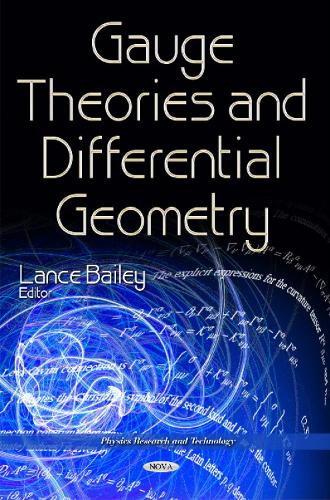•# Gauge Theories & Differential Geometry (Hardback)

(editor)
£180.00
Hardback 278 Pages / Published: 01/12/2015
• We can order this

Usually dispatched within 3 weeks

This book revisits the mathematical foundations of thermodynamics and gauge theory by using new differential geometric methods coming from the formal theory of systems of partial differential equations and Lie pseudogroups. The gauge theory of gravity is also established, in which spinorial and ventorial matter fields serve as gravitating sources. The potential applications of the present gauge theory of gravity, including quantum-vacuum-energy gravity, cosmological constant problem and gravity-gauge unification is also addressed. The third chapter focuses on a gravitational gauge theory with spin connection and vierbein as fundamental variables of gravity. Next, the place and physical significance of Poincare gauge theory of gravity (PGTG) in the framework of gauge approach to gravitation is discussed. A cutoff regularization method in gauge theory is discussed in Chapter Five. The remaining chapters in the book focus on differential geometry, in particular, the authors show how fractional differential derived from fractional difference provides a basis to expand a theory of fractional differential geometry which would apply to non-differentiable manifolds; a review of the infinitesimal Baker-Campbell-Hausdorff formula is provided and the book concludes with a short communication where the authors focus on local stability, and describe how this leads naturally into the question of finite-time singularities and generalized soliton solutions.

Publisher: Nova Science Publishers Inc
ISBN: 9781634835466
Number of pages: 278
Weight: 742 g
Dimensions: 260 x 180 mm